# Controller Design Approach for SVPWM-Regulated AC/DC Rectifier

Controller Design Approach for SVPWM-Regulated AC/DC Rectifier
Research & Innovation Department, Capgemini Engineering, Direction Research & Innovation Department, 96 avenue Charles de Gaulle, Neuilly-sur-Seine 92200, France

National Higher School of Electrical Engineering, Electronics, Computing, Hydraulics and Telecommunications (ENSEEIHT), 2 Rue Charles Camichel, Toulouse 31000, France

Corresponding Author Email:
naziha.harrabi@capgemini.com
Page:
353-360
|
DOI:
https://doi.org/10.18280/ejee.230501
7 October 2021
|
Accepted:
23 October 2021
|
Published:
31 October 2021
| Citation

OPEN ACCESS

Abstract:

Electric vehicles (EVs) are widely used all over the world and has been attracting increasing attention. The grid-connected AC/DC rectifier is one of the main parts in the EV battery charger. It can realize the power flow and meet the power quality requirements for grid. Therefore, a three-phase bidirectional grid-connected AC/DC converter is studied in this paper for batteries charging system. First, the configuration of the system is introduced and the mathematical model of the converter is built. Then, we present, for the AC/DC converter, the model simulation of Space Vector Pulse Width Modulation (SVPWM) for different use cases using Matlab/SIMULINK software. The objective of the proposed model is to stabilize the output voltage from a three phase system using a decoupling control strategy based on direct quadrature (d-q) frame method. The designed control scheme is tested for two cases of study. Simulation results show that the SVPWM control applied to the rectifier has an adequate performance and can ensure the control objectives effectively.

Keywords:

SVPWM control, AC/DC converter, rectifier, decoupling control, three phase system

1. Introduction

Electric Vehicles (EVs) have attracted an important attention worldwide due to their numerous advantages such as environment protection and low cost. In the charging or discharging mode of the EVs batteries, AC/DC converters have a key role in the power flow and quality. In recent years, a considerable increase has been put on the AC/DC converter topologies used in EVs applications in order to provide a fast dynamic response and low output voltage distortion. High efficiency and Low Total Harmonic Distortion (THD) are actually very required in all high power applications like three-phase systems.

Due to several reasons, such as the low cost of power semiconductors and control circuits, Pulse Width Modulation (PWM) based AC-DC converters were among the most used AC-DC converters . According to the existing literature, various PWM modulation techniques can be used to control AC-DC converters. The main control objective is to get a variable voltage and frequency supply. The most popular methods are sinusoidal, delta, and space vector modulation methods [2, 3].

Space Vector Pulse Width Modulation (SVPWM) technique is known as a powerful control strategy. This latter is a popular method for the control of motor drives and three-phase rectifiers. It has the advantage of ensuring less voltage/current harmonics, high use rate of DC voltage, and less output waveform distortion when compared to Sine-wave Pulse Width Modulation (SPWM) method. In fact, the principal advantage of SVPWM over SPWM is that it enhances the DC bus use by about 15%.

In summary, this method provides reduced switching losses and better DC bus utilization compared to conventional PWM modulation [4, 5].

To the best of our knowledge, most of the existing work does not provide a systematic approach for controller design and implementation of SVPWM control strategy for AC/DC converters. Therefore, the main idea of this paper is to design an SVPWM controller for an AC/DC converter. Several use cases were considered to evaluate the effectiveness of the proposed controller using simulation performed in Matlab/SIMULINK.

The remainder of this paper can be outlined as follows: a mathematical model of the proposed system including three-phase PWM rectifier, the load and the filter as described in section 2; in section 3, the SVPWM algorithm is described; the current and voltage control loops are presented in section 4; the obtained simulation results are reported and discussed in section 5, and followed by a brief summary in section 6.

2. Modeling of the System

The system under consideration in this work is shown in Figure 1. It shows the topology of a three-phase voltage-source AC-DC converter.

The converter is connected to the grid through an R-L filter, while the DC-side consists of a DC-link and resistive loads. The AC grid source is denoted as $e_{a}, e_{b}, e_{c}$ and $i_{a}, i_{b}, i_{c}$ denote the source current. L represents the inductance filter, and $R$ is the resistance of series $R-L$ circuit. $C$ denotes the DC-side capacitor. $V_{d c}$ and $I_{d c}$ are respectively the DC-side voltage and current. Two resistances in parallel are equivalent to the DC load.

## 1.png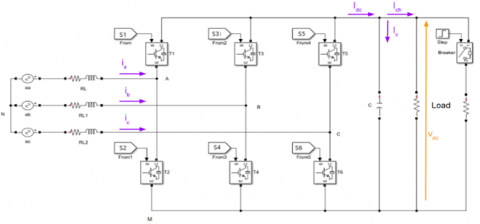Figure 1. Circuit schematic of the three phase grid-side AC/DC converter

Analytical model is an important step for the prediction of dynamic performance and stability limits using different control laws and system parameters. Mathematical model of the rectifier must be established before the design stage.

The equations representation of the grid three phases voltages is given as following [6, 7]:

$\left[\begin{array}{l}e_{a} \\ e_{b} \\ e_{c}\end{array}\right]=R\left[\begin{array}{c}i_{a} \\ i_{b} \\ i_{c}\end{array}\right]+L \frac{d}{d t}\left[\begin{array}{l}i_{a} \\ i_{b} \\ i_{c}\end{array}\right]+\left[\begin{array}{l}V_{A M} \\ V_{B M} \\ V_{C M}\end{array}\right]+\left[\begin{array}{c}V_{A N} \\ V_{B N} \\ V_{C N}\end{array}\right]$      (1)

where, $e_{a, b, c}$ and $i_{a, b, c}$ are respectively phase voltages and currents, $V_{A M, B M, C M}$ are voltages between nodes $(\mathrm{A}, \mathrm{B}, \mathrm{C})$ and $\mathrm{M}$, and $V_{A N, B N, C N}$ are voltages between nodes $(\mathrm{A}, \mathrm{B}, \mathrm{C})$ and $\mathrm{N}$ (neutral of the three phase source).

The current in the DC link is expressed as following:

$I_{d c}=C \frac{d V_{d c}}{d t}+\frac{V_{d c}}{R}$      (2)

where, C represents the value of the DC bus filter capacitor and $\mathrm{R}$ the resistive load. $V_{d c}$ is the voltage of the $\mathrm{DC}$ bus.

For simplifying purposes, the system model can be transformed into the $\mathrm{d}$ - $\mathrm{q}$ synchronously rotating reference frame at the utility frequency $\omega$, by using Park's transformation. Consequently, the obtained dynamic model is given by:

$\left\{\begin{array}{l}e_{d}=R i_{d}+L \frac{d i_{d}}{d t}-L \omega i_{q}+V_{d} \\ e_{q}=R i_{q}+L \frac{d i_{q}}{d t}-L \omega i_{q}+V_{d}\end{array}\right.$       (3)

where, $\left(e_{d}, e_{q}\right)$ and $\left(i_{d}, i_{q}\right)$ represent respectively voltages and currents components in $\mathrm{d}-\mathrm{q}$ frame and $\left(V_{d}, V_{q}\right)$ are the $\mathrm{d}-$ axis and $\mathrm{q}$-axis components of the rectifier $\mathrm{AC}$ voltages.

3. SVPWM Control

The space vector modulation technique is a variation of the pulse width modulation control. It is based on the vector representation of voltages in the complex plane.

The basis of SVPWM is the principle of average value equivalence, that is, the basic voltage vector is combined, within a switching period, to make switching cycle to make its average value equal to the given voltage vector .

The circuit schematic of the above-mentioned converter is shown in Figure 1. It is a three-phase converter composed of six PWM-controlled switches (i.e. S1, S2..., S6).

In the converter circuit shown above, we set the voltage on the DC bus to $V_{d c}$. Three-phase voltages are applied to a plane coordinate system and they are phase-shifted by 120 degrees in space.

By defining these three voltage-space vectors as: $\vec{V}_{A N}(t)$, $\vec{V}_{B N}(t)$ and $\vec{V}_{C N}(t)$, their directions are always on their respective axes, while the size changes in time according to the law of sine, and the time phases is 120 degrees.

Assuming that $V_{m}$ is the maximum value of the phase voltage and f is the power frequency, then for an ideal three-phase voltage we have:

$\left\{\begin{array}{c}\vec{V}_{A N}(t)=V_{m} \cos (\omega t) \\ \vec{V}_{B N}(t)=V_{m} \cos \left(\omega t-\frac{2 \pi}{3}\right) \\ \vec{V}_{C N}(t)=V_{m} \cos \left(\omega t-\frac{4 \pi}{3}\right)\end{array}\right.$     (4)

The SVPWM technique is mainly based on the determination of a space vector, namely $V_{r e f}(t)$, in the plane $(\alpha, \beta)$ which is equivalent to the target three phase output voltage system.

$\vec{V}_{r e f}(t)=\frac{2}{3}\left(\vec{V}_{A N}(t)+\vec{V}_{B N}(t) e^{j \frac{2 \pi}{3}}+\vec{V}_{C N}(t) e^{j \frac{4 \pi}{3}}\right)$      (5)

$\vec{V}_{r e f}(t)$ is a rotating space vector where the amplitude is constant and equals to the phase peak voltage, and the angular frequency $\omega=2 \pi f$ where $f$ is the frequency. Space vector rotating at a constant speed in a counterclockwise direction. The SVPWM purpose is to use the switching state of the threephase bridge to represent $\vec{V}_{r e f}(t)$.

For a three-phase system, the input voltages $\left(V_{A N}, V_{B N}, V_{C N}\right)$ are defined from the states of switches $\mathrm{S} 1, \mathrm{~S} 3$ and $\mathrm{S} 5$ ( $\mathrm{S} 2, \mathrm{~S} 4$ and $\mathrm{S} 6$ take the complementary states), following this equation:

$V_{r e f}=\frac{2}{3} V_{d c}\left(S_{A}+S_{B} e^{j \frac{2 \pi}{3}}+S_{C} e^{j \frac{4 \pi}{3}}\right)$     (6)

$\left[\begin{array}{l}V_{A N} \\ V_{B N} \\ V_{C N}\end{array}\right]=\frac{V_{d c}}{3}\left[\begin{array}{ccc}2 & -1 & -1 \\ -1 & 2 & -1 \\ -1 & -1 & 2\end{array}\right]\left[\begin{array}{l}S_{1} \\ S_{2} \\ S_{3}\end{array}\right]$      (7)

Table 1. Phase voltage values for the different switching state of the three-phase rectifier

 Vectors Switches states Phase Voltage A B C $V_{A N}$ $V_{B N}$ $V_{C N}$ 0 0 0 0 0 0 0 1 1 0 0 $\frac{2 V_{d c}}{3}$ $\frac{-V_{d c}}{3}$ $\frac{-V_{d c}}{3}$ 2 1 1 0 $\frac{V_{d c}}{3}$ $\frac{V_{d c}}{3}$ $\frac{-2 V_{d c}}{3}$ 3 0 1 0 $\frac{-V_{d c}}{3}$ $\frac{2 V_{d c}}{3}$ $\frac{-V_{d c}}{3}$ 4 0 1 1 $-\frac{2 V_{d c}}{3}$ $\frac{V_{d c}}{3}$ $\frac{V_{d c}}{3}$ 5 0 0 1 $\frac{-V_{d c}}{3}$ $\frac{-V_{d c}}{3}$ $\frac{2 V_{d c}}{3}$ 6 1 0 1 $\frac{V_{d c}}{3}$ $\frac{-2 V_{d c}}{3}$ $\frac{V_{d c}}{3}$ 7 1 1 1 0 0 0

Since the three-phase bridge arm of the converter has a total number of 6 switches (S1, S2...S6), we have to study the space voltage vector for different switch combinations of upper and lower bridge arms of each phase. We can identify eight different possibilities as explained in Table 1.

According to Table 1, the three-phase rectifier can be working in eight different modes (000~111), including six non-zero vectors and two zero vectors (000,111).

By putting the above 8 voltage space vectors according to the output, the phase relationship is placed in the sector diagram.

In Figure 2, the six non-zero vectors have the same amplitude, and the adjacent vectors are phase-shifted by 60 degrees each [8-10]. The amplitude of the two zero vectors is zero, which are located in the center.

## 2.png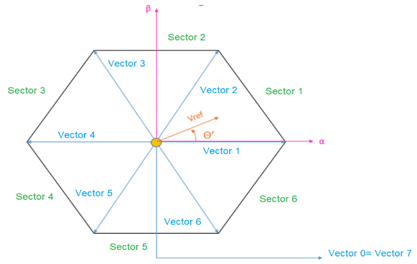Figure 2. Available switching vectors and sector definition of the three-phase rectifier

Table 2 presents the switching sequences for the lower and upper switches, IGBTs in our case.

Table 2. The three-phase rectifier switching sequence table

 Sector Upper switches (S1, S3, S5) Lower switchers (S2, S4, S6) 1 $S_{1}=T_{1}+T_{2}+T_{0} / 2$ $S_{3}=T_{2}+T_{0} / 2$ $S_{5}=T_{0} / 2$ $S_{4}=T_{0} / 2$ $S_{6}=T_{1}+T_{0} / 2$ $S_{2}=T_{1}+T_{2}+T_{0} / 2$ 2 $S_{1}=T_{1}+T_{0} / 2$ $S_{3}=T_{1}+T_{2}+T_{0} / 2$ $S_{5}=T_{0} / 2$ $S_{4}=T_{2}+T_{0} / 2$ $S_{6}=T_{0} / 2$ $S_{2}=T_{1}+T_{2}+T_{0} / 2$ 3 $S_{1}=T_{0} / 2$ $S_{3}=T_{1}+T_{2}+T_{0} / 2$ $S_{5}=T_{2}+T_{0} / 2$ $S_{4}=T_{1}+T_{2}+T_{0} / 2$ $S_{6}=T_{0} / 2$ $S_{2}=T_{1}+T_{0} / 2$ 4 $S_{1}=T_{0} / 2$ $S_{3}=T_{1}+T_{0} / 2$ $S_{5}=T_{1}+T_{2}+T_{0} / 2$ $S_{4}=T_{1}+T_{2}+T_{0} / 2$ $S_{6}=T_{2}+T_{0} / 2$ $S_{2}=T_{0} / 2$ 5 $S_{1}=T_{2}+T_{0} / 2$ $S_{3}=T_{0} / 2$ $S_{5}=T_{1}+T_{2}+T_{0} / 2$ $S_{4}=T_{1}+T_{0} / 2$ $S_{6}=T_{1}+T_{2}+T_{0} / 2$ $S_{2}=T_{0} / 2$ 6 $S_{1}=T_{1}+T_{2}+T_{0} / 2$ $S_{3}=T_{0} / 2$ $S_{5}=T_{1}+T_{0} / 2$ $S_{4}=T_{0} / 2$ $S_{6}=T_{1}+T_{2}+T_{0} / 2$ $S_{2}=T_{2}+T_{0} / 2$

To simplify the space vector PWM, the voltage conditions in the $(\mathrm{a}, \mathrm{b}, \mathrm{c})$ reference frame can be transformed into the stationary $(\mathrm{d}, \mathrm{q})$ reference frame as drew in Figure 3 and expressed by Eq. (8). Hence, the vector $\vec{V}_{r e f}=V e^{j \theta^{\prime}}$, its module $V$ and its angle $\theta^{\prime}$ can be determined as following:

$\vec{V}_{r e f}=\left(\begin{array}{c}\vec{V}_{\alpha} \\ \vec{V}_{\beta}\end{array}\right)=\frac{2}{3}\left[\begin{array}{ccc}1 & -\frac{1}{2} & -\frac{1}{2} \\ 0 & \frac{\sqrt{3}}{2} & -\frac{\sqrt{3}}{2}\end{array}\right]\left[\begin{array}{l}\vec{V}_{A N} \\ \vec{V}_{B N} \\ \vec{V}_{C N}\end{array}\right]$     (8)

$V=\sqrt{V_{\beta}^{2}+V_{\alpha}^{2}}$      (9)

$\theta^{\prime}=\arctan \left(\frac{V_{\beta}}{V_{\alpha}}\right)$      (10)

The repartition of sectors, illustrated in Figure 3, is defined by the following conditions:

Sector 1: if $0<\theta^{\prime}<\frac{\pi}{3}$

Sector 2: if $\frac{\pi}{3}<\theta^{\prime}<\frac{2 \pi}{3}$

Sector 3: if $\frac{2 \pi}{3}<\theta^{\prime}<\pi$

Sector 4: if $-\pi<\theta^{\prime}<-\frac{2 \pi}{3}$

Sector 5: if $-\frac{2 \pi}{3}<\theta^{\prime}<-\pi$

Sector 6: if $-\frac{\pi}{3}<\theta^{\prime}<-\pi$

In order to obtain a fixed switching frequency and optimum harmonic performance from SVPWM, each leg should change its sate only once in one switching period. This is achieved by applying zero state vector followed by two adjacent active state vector in half switching period. The next half of the switching period is the mirror image of the first half. The total switching period is divided into 7 parts, the zero vector is applied for 1/4th of the total zero vector time first followed by the application of active vectors for half of their application time and then again zero vector is applied for 1/4th of the zero vector time. This is then repeated in the next half of the switching period. In a way to obtain symmetrical SVPWM.

$\left\{\begin{array}{c}T_{0}=T_{7} \\ T_{0}+T_{1}+T_{2}+T_{7}=T_{d e c}\end{array} \rightarrow T_{0}=\frac{T_{d e c}-T_{1}-T_{2}}{2}\right.$      (11)

where, $T_{\text {dec }}$ is the decoupling period of time.

By admitting that $V_{d c}=E$, based on , and combining Eqns. (5) and (11), we obtain the following equation:

$V e^{j \theta \prime}=\frac{T_{1}}{T_{d e c}} \frac{2}{3} E+\frac{T_{2}}{T_{d e c}} \frac{2}{3} E e^{j \pi / 3}$      (12)

Hence, the time duration of active space voltage vectors is computed as follows:

$\left\{\begin{array}{c}T_{2}=\sqrt{3} T_{d e c} \frac{|V|}{E} \sin \left(\theta^{\prime}\right) \\ T_{1}=\sqrt{3} T_{d e c} \frac{|V|}{E} \cos \left(\theta^{\prime}+\frac{\pi}{6}\right) \\ T_{0}=\frac{T_{d e c}}{2}\left(1-\frac{\sqrt{3}|V|}{E}\right) \cos \left(\theta^{\prime}-\frac{\pi}{6}\right)\end{array}\right.$      (13)

The same procedure can be followed to calculate the vector's duration for all sectors. Once the active vector is calculated the time durations can be calculated.

## 3.png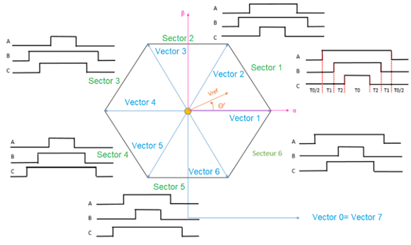Figure 3. Space vector modulation with response in each rectifier leg

4. Current and Voltage Control

The control objective for the studied three-phase rectifier is to generate sinusoidal input phase-synchronized currents with the input voltages and to regulate the DC output voltage.

In this strategy of control, both the voltage and current signals should be measured. The corrected voltage and the output voltage of the error intensifier are duplicated and can give the reference current. The control is based on the following equations in d-q frame:

$\left\{\begin{array}{l}e_{d}=R i_{d}+L \frac{d i_{d}}{d t}-L \omega i_{q}+V_{d} \\ e_{q}=R i_{q}+L \frac{d i_{q}}{d t}-L \omega i_{q}+V_{d}\end{array}\right.$      (14)

The desired signals of voltage are expressed by:

$V_{d}{ }^{*}=e_{d}-\left(K_{p}+\frac{K_{i}}{s}\right)\left(i_{d}{ }^{*}-i_{d}\right)+\omega L i_{q}$      (15)

$V_{q}{ }^{*}=e_{q}-\left(K_{p}+\frac{K_{i}}{s}\right)\left(i_{q}{ }^{*}-i_{q}\right)-\omega L i_{q}$      (16)

4.1 Current control

The function of the current loops PI controllers is to produce the voltage commands signals for the SVPWM modulator block.

We consider the simplified transfer function:

$T(p)=\frac{1}{L p}$      (17)

The PI controller transfer function has the following form:

$C_{d}(p)=C_{q}(p)=\left(\frac{K T_{i} p+K}{T_{i} p}\right)$       (18)

where the parameters of the controller are calculated as follows:

$K=L \omega_{b p}$: the gain;

$T_{i}=\frac{\sqrt{10}}{\omega_{b p}}$: the time constant;

$\omega_{b p}=\frac{\omega_{d e c}}{10}$: the pass band (for a phase margin $\mathrm{PM}=55^{\circ}$).

4.2 Voltage control

In the previous paragraph, we presented the current regulation loop necessary to absorb a sinusoidal input current in order to absorb only active power while setting the reactive power to zero. This allows to have an active power proportional to the magnitude of the current.

In this part, we will present the voltage regulation, which is related to the previous current control. In fact, the output voltage controller generates the reference current of the d axis, which will be injected into the current regulation loop. This reference current allows obtaining an output voltage equals to the desired value. For the voltage regulation loop, based on the power balance, we can define the transfer function on the basis of the following equations:

$\left\langle P_{i n}\right\rangle=V_{d} i_{d}=\left\langle V_{D C}\right\rangle\left\langle i_{D C}\right\rangle$     (19)

where, $P_{i n}$ represents the input Power. By substituting $i_{d}$ by its expression, we get:

$\frac{V_{d} i_{D C}}{2\left\langle V_{D C}\right\rangle}=C \frac{d\left\langle V_{D C}\right\rangle}{d t}+\frac{\left\langle V_{D C}\right\rangle}{R}$      (20)

By developing the last equation, we obtain:

$\frac{V_{d} i_{r e f}}{2}=C \frac{\left.d<V_{D C}^{2}\right\rangle}{d t}+\frac{\left\langle V_{D C}^{2}\right\rangle}{R}$      (21)

We suppose that $V_{D C}^{2}=X$, then (21) becomes:

$\frac{V_{d} i_{r e f}}{2}=C \frac{d X}{d t}+\frac{X}{R}$       (22)

In Laplace domain, we can write:

$\frac{X(p)}{i_{d}(p)}=\frac{R V_{d}}{R C p+1}$       (23)

The controller transfer function form is then given by:

$\mathrm{C}(p)=\left(\frac{K T_{i} p+K}{T_{i} p}\right)$      (24)

Figure 4 illustrates the bloc-schematic of the overall controller (for current and voltage regulation).

The actual DC voltage is, first, compared with the reference voltage, and the difference between these two voltages is fed to the voltage PI controller. Then, the output of this latter is transmitted to the current controller block. The current PI controller aims to minimize the error between the d- axis current and its reference value.

The output of the current controller is then fed to the transformation block. Similarly, the q- axis actual input is compared with the desired value and the error is then minimized by the PI controller. These two quantities, Vd_control and Vq_control, are given to the space vector modulation block in order to generate the control signals of the three-phase rectifier.

## 4.png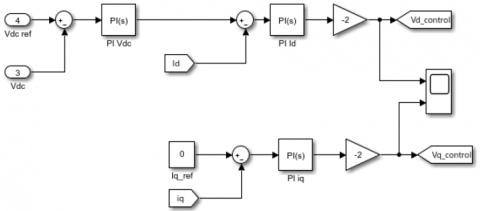Figure 4. Voltage and current control scheme

## 5.pngFigure 5. The used simulation scheme

5. Simulations Results

Simulations are mainly performed, in power electronic systems, in order to design and evaluate the circuit configuration and the applied control strategy effectiveness.

The studied model has been simulated under Matlab SIMULINK software to verify the operation of the proposed control method. Figure 5 shows the used simulation scheme. It includes the AC/DC converter connected to three-phase source and a variable DC load.

Two cases studies have been presented and analysed in order to check the efficiency of the proposed control system. We start by analyzing the first case that corresponds to 230 V AC source and 400 V DC output.

Simulations were carried out applying parameters values which are shown in Table 3.

Table 3. System characteristics

 First case Second case Nominal power Three phase Voltage DC Bus voltage Ripple on output voltage Grid frequency Decoupling frequency 10 kW 230 V 400 V 10% 50 Hz 9 kHz 10 kW 400 V 800 V 10% 50 Hz 9 kHz

## 6.png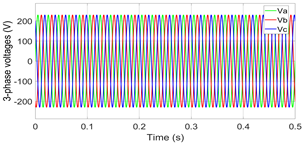Figure 6. Three-phase source's voltages

## 7.png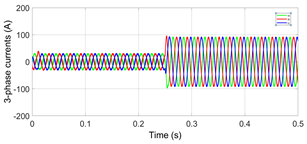Figure 7. Three-phase input's currents

## 8.png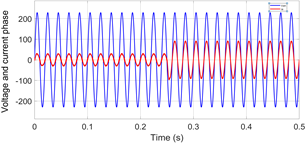Figure 8. Input voltage and current of the three -phase AC-DC converter

## 9.png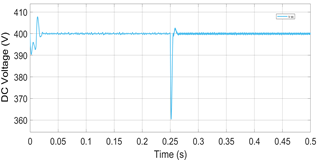Figure 9. DC bus voltage variation

## 10.png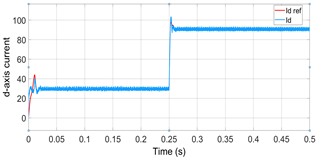Figure 10. d-axis current component and its reference

## 11.png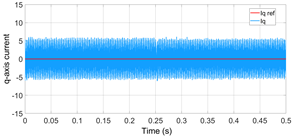Figure 11. q-axis current component and its reference

The simulation results of the second study case corresponds to a 400V AC source and 800V DC output are presented as follows.

## 12.pngFigure 12. Three phase voltages source

## 13.png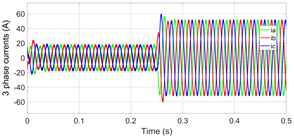Figure 13. Three phase currents source

## 14.png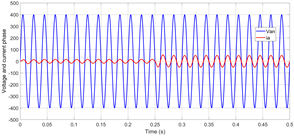Figure 14. Input voltage and current of three -phase AC to DC converter with PI controllers

## 15.pngFigure 15. DC bus voltage

## 16.pngFigure 16. d-axis current component and its reference

## 17.png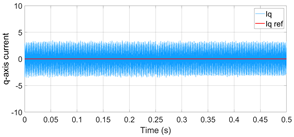Figure 17. q-axis current component and its reference

Figures 6 and 7 represent, respectively, the three-phase currents and voltages of the source.

Figure 8 shows the input voltage and current waveform of the three-phase AC/ DC controlled. It can be seen that the voltage and the current are synchronous and have the same phase. The controller can ensure robust results although the load variation applied at t=0.25 s.

Figure 9 shows the output voltage waveform in the DC bus which is regulated around the desired value for both of study cases. We can remark that there is a ripple at the time of load variation application but this oscillation is accepted and do not exceed 10% of the nominal voltage value. The same remarks can be concluded when analyzing Figure 15 that is related for the second case of study.

The controlled current components in d axis and q axis for the first case of study are respectively shown by Figures 10 and 11.

For the second case of simulation, the three-phase voltages and currents source are shown, respectively, in Figures 12 and 13. However, Figure 14 represents the input voltage and current of the three -phase AC /DC converter controlled with PI controllers.

Similarly, controlled current components in d axis and q axis for the second case of study are respectively shown by Figures 16 and 17. Controlled actual signals ($i_{d}$ and $i_{q}$) are tracking the desired signals ($i_{\text {dref }}$ and $i_{\text {qref }}$) as can be seen from the graphics, which prove that SVPWM has good drive response if we have load variation.

6. Conclusions

This work presents a three-phase grid-connected AC/DC converter for EV batteries charging system. The mathematic model of the three-phase rectifier in the synchronous d-q frame is built and SVPWM controller is elaborated. The converter control was examined in various use cases by varying the desired outputs. These tests were realized utilizing different control systems, designed with PI controllers of voltage and current closed loop. Finally, the model is established using Matlab SIMULINK in order to evaluate the proposed control strategy. The overall performance for the proposed control strategy is great and ensure robust results against load variations. In summary, the fixed control objectives were ensured by applying the proposed control.

Acknowledgment

This work was supported by Altran Prototype Automobile, Research & Innovation Department, Capgemini-Engineering and realized under research internship for Swap Technology (SawpTech) project. Authors would like also to thank other team members Guillaume Voizard, Michel Geahel and Frédéric Guimard for their assistance and support.

Nomenclature
 SVPWM Space Vector Pulse Width Modulation IGBT Insulated Gate Bipolar Transistor EV Electric Vehicle THD Total Harmonic Distortion
References

 Undeland, M.N., Robbins, W.P., Mohan, N. (1995). Power electronics. In Converters, Applications, and Design. John Whiley & Sons.

 Monroy-Morales, J.L., Campos-Gaona, D., Hernández-Ángeles, M., Peña-Alzola, R., Guardado-Zavala, J.L. (2017). An active power filter based on a three-level inverter and 3D-SVPWM for selective harmonic and reactive compensation. Energies, 10(3): 297. http://doi.org/10.3390/en10030297

 Khan, M.Z., Naveed, M.M., Hussain, D.A. (2013). Three phase six-switch PWM buck rectifier with power factor improvement. In Journal of Physics: Conference Series, 439: 012028. http://doi.org/10.1088/1742-6596/439/1/012028

 Fadel, M. (2019). Modélisation et Commande Avancée des Convertisseurs Statiques. ENSEEIHT, Toulouse.

 Krishna, K.G., Kumar, T.K., Rao, P.V. (2012). Better DC bus utilization and torque ripple reduction by using SVPWM for VSI fed induction motor drive. International Journal of Computer and Electrical Engineering, 4(2): 202-206202. http://doi.org/10.7763/IJCEE.2012.V4.479

 Van Nho, N. (2005). A graphical approach to switching losses and harmonics distortion for carrier SVPWM methods in multilevel inverters. In 2005 International Conference on Power Electronics and Drives Systems, pp. 192-197. http://doi.org/10.1109/PEDS.2005.1619684

 Marroyo, L., Piquet, H., Richardeau, F. (1998). Méthodologie de dimensionnement d'un redresseur MLI à prélèvement sinus. In Conference: Electronique de Puissance du Futur EPF'1998.

 Sarkar, P., Bhunia, S. (2017). SVPWM based vector control of PMSM drive in delta domain. In 2017 International Conference on Electrical, Computer and Communication Engineering (ECCE), pp. 5-10. http://doi.org/10.1109/ECACE.2017.7912869

 Holmes, D.G. (1992). The general relationship between regular-sampled pulse-width-modulation and space vector modulation for hard switched converters. In Conference Record of the 1992 IEEE Industry Applications Society Annual Meeting, pp. 1002-1009. http://doi.org/10.1109/IAS.1992.244437

  Kumar, A., Chatterjee, D. (2017). A survey on space vector pulse width modulation technique for a two-level inverter. In 2017 National Power Electronics Conference (NPEC), pp. 78-83. http://doi.org/10.1109/NPEC.2017.8310438

 Schönberger, J. (2015). Space vector control of a three-phase rectifier using PLECS. Plexim GmbH. [Online]. Available: http://www.plexim.com/sites/default/files/plecs_svm.pdf., accessed on 22 March 2016.